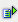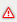The function code (string) returns the ASCII code of the first character in the text string.

Syntax: =code(string)Example =code("z") is 122 =code(zenon) is 122 If the cell A1 contains the string zenon the function =code (A1) returns the value 122 as a result.Attention The function code (string) returns the ASCII code of the first character in the text string.